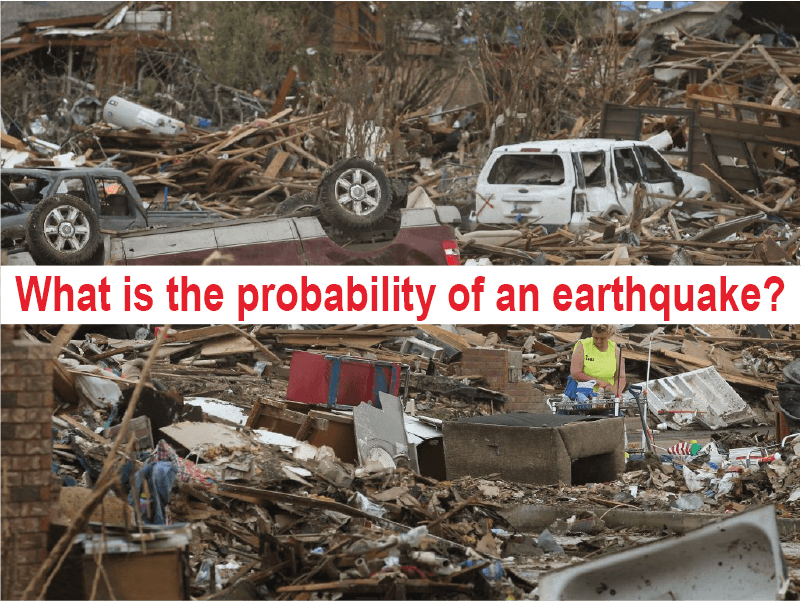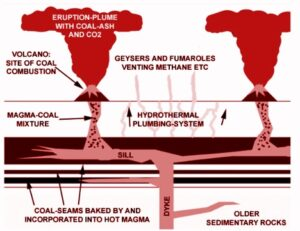January 28, 2023

# DRE 421 (Earthquake Engineering and Management)The probability of an earthquake is something that has to be related to a region. For example, what is the probability of an earthquake occurring in Japan or USA or Bangladesh or Sri Lanka. The answer depends on the regional tectonics.  I will take two examples. Japan and Sri Lanka (I don’t know about Bangladesh). So here Japan is a country that is on the boundary of convergence of two plates, one sub-ducting to the other. In Japan’s case, the Pacific plate is sub-ducting below the Eurasian plate. In such an environment there are many earthquakes happening and the probability is quite high and the calculation of happening an earthquake is specific to one another.

But in Sri Lanka the tectonic environment is very dull. there are no plates colliding in that zone and thus the probability of an earthquake is very low.

Now, back to Japan. I said that the probability of one happening is quite high, but this is only for small earthquakes.

Ex. even though we cannot feel there are earthquakes happening all the time which are of magnitudes 1,2 or 3.

But the ones higher than that are not so often they are happening with a certain interval. which is called the recurrence time.

So depending on the recurrence interval the probability of one happening is different.

For small earthquakes the recurrence interval is small but for very large earthquakes that is high.

therefore the probability of a small earthquake is large and the probability of a large earthquake is small.

The probability can be calculated for a given period using historical data. For a given time, say for 100 years, see the total number of earthquakes that has happened, Say 1000. Now among them how many earthquakes are there with the magnitude 5.5 take them, say 500. Then the probability of an earthquake happening with a magnitude of 5.5 is 500/1000 which is 1/2 for 100 years.

Then consider an earthquake happening with a magnitude 7.5 occurring in the same period, say the number is 50. The probability of that is 50/1000, that is 1/20.

Then consider an earthquake with a size of 9.4 (As the Miyagi-oki eq,2011). These are very rare. For the considered same period the number of these kind of earthquakes are very small, say 1. Then the probability of that happening is 1/1000.

So like this the probability of an earthquake is something that is limited to a region. But if you consider the whole world and do the calculations then also you can get a number. But it is not very specific.

Therefore as I said the probability of an earthquake is specific to a region.

This is a very big area. many things are taken into consideration when you in do details and calculating the probabilities. The historical data, the rate of plate subduction, the strain rate calculations, the morphology of the subducting plate, like this there are many things that are needed to be considered.

But even after such an effort, an earthquake can happen out of no where.

Ex. the one that happened in Fukushima couple of weeks ago. Scientist considered that to be an aseismic region (area that earthquakes cannot occur), but out of blue this happens and every body is shoked.

This is why it is so difficult to predict earthquakes and the probabilities.

Do you have more question? Hope you found some answer from bellow.

## Assignments For students

Assignment on EQ Super cycle (Solved)

#### You may have missed#### Causes of Permian Mass extinction